# Title 40

## SECTION 1065.640

### 1065.640 Flow meter calibration calculations.

§ 1065.640 Flow meter calibration calculations.

This section describes the calculations for calibrating various flow meters. After you calibrate a flow meter using these calculations, use the calculations described in § 1065.642 to calculate flow during an emission test. Paragraph (a) of this section first describes how to convert reference flow meter outputs for use in the calibration equations, which are presented on a molar basis. The remaining paragraphs describe the calibration calculations that are specific to certain types of flow meters.

(a) Reference meter conversions. The calibration equations in this section use molar flow rate, n ref, as a reference quantity. If your reference meter outputs a flow rate in a different quantity, such as standard volume rate, v stdref, actual volume rate, v actref, or mass rate, m ref, convert your reference meter output to a molar flow rate using the following equations, noting that while values for volume rate, mass rate, pressure, temperature, and molar mass may change during an emission test, you should ensure that they are as constant as practical for each individual set point during a flow meter calibration: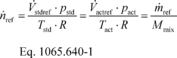Where: n ref = reference molar flow rate. v stdref = reference volume flow rate, corrected to a standard pressure and a standard temperature. v actref = reference volume flow rate at the actual pressure and temperature of the flow rate. m ref = reference mass flow. pstd = standard pressure. pact = actual pressure of the flow rate. Tstd = standard temperature. Tact = actual temperature of the flow rate. R = molar gas constant. Mmix = molar mass of the flow rate. Example 1: v stdref = 1000.00 ft 3/min = 0.471948 m 3/s pstd = 29.9213 in Hg @32 °F = 101.325 kPa = 101325 Pa = 101325 kg/(m·s 2) Tstd = 68.0 °F = 293.15 K R = 8.314472 J/(mol·K) = 8.314472 (m 2·kg)/(s 2·mol·K)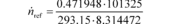n ref = 19.619 mol/s Example 2: m ref = 17.2683 kg/min = 287.805 g/s M mix = 28.7805 g/mol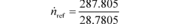n ref = 10.0000 mol/s

(b) PDP calibration calculations. Perform the following steps to calibrate a PDP flow meter:

(1) Calculate PDP volume pumped per revolution, Vrev, for each restrictor position from the mean values determined in § 1065.340 as follows: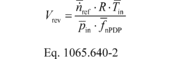Where: n ref = mean reference molar flow rate. R = molar gas constant. T in = mean temperature at the PDP inlet. P in = mean static absolute pressure at the PDP inlet. f nPDP = mean PDP speed. Example: n ref = 25.096 mol/s R = 8.314472 J/(mol·K) = 8.314472 (m 2·kg)/(s 2·mol·K) T in = 299.5 K P in = 98.290 kPa = 98290 Pa = 98290 kg/(m·s 2) f nPDP = 1205.1 r/min = 20.085 r/s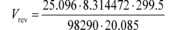Vrev = 0.03166 m 3/r

(2) Calculate a PDP slip correction factor, Ks, for each restrictor position from the mean values determined in § 1065.340 as follows: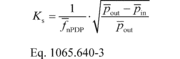Where: f nPDP = mean PDP speed. P out = mean static absolute pressure at the PDP outlet. P in = mean static absolute pressure at the PDP inlet. Example: f nPDP = 1205.1 r/min = 20.085 r/s P out = 100.103 kPa P in = 98.290 kPa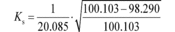Ks = 0.006700 s/r

(3) Perform a least-squares regression of Vrev, versus Ks, by calculating slope, a1, and intercept, a0, as described in § 1065.602.

(4) Repeat the procedure in paragraphs (b)(1) through (3) of this section for every speed that you run your PDP.

(5) The following table illustrates a range of typical values for different PDP speeds:

Table 1 of § 1065.640 - Example of PDP Calibration Data

f nPDP
(revolution/s)
a1
(m 3/s)
a0
(m 3/revolution)
12.6 0.841 0.056
16.5 0.831 −0.013
20.9 0.809 0.028
23.4 0.788 −0.061

(6) For each speed at which you operate the PDP, use the appropriate regression equation from this paragraph (b) to calculate flow rate during emission testing as described in § 1065.642.

(c) Venturi governing equations and permissible assumptions. This section describes the governing equations and permissible assumptions for calibrating a venturi and calculating flow using a venturi. Because a subsonic venturi (SSV) and a critical-flow venturi (CFV) both operate similarly, their governing equations are nearly the same, except for the equation describing their pressure ratio, r (i.e., rSSV versus rCFV). These governing equations assume one-dimensional isentropic inviscid flow of an ideal gas. Paragraph (c)(5) of this section describes other assumptions that may apply. If good engineering judgment dictates that you account for gas compressibility, you may either use an appropriate equation of state to determine values of Z as a function of measured pressure and temperature, or you may develop your own calibration equations based on good engineering judgment. Note that the equation for the flow coefficient, Cf, is based on the ideal gas assumption that the isentropic exponent, g, is equal to the ratio of specific heats, Cp/Cv. If good engineering judgment dictates using a real gas isentropic exponent, you may either use an appropriate equation of state to determine values of γ as a function of measured pressures and temperatures, or you may develop your own calibration equations based on good engineering judgment.

(1) Calculate molar flow rate, n , as follows: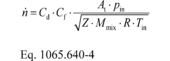Where:

Cd = discharge coefficient, as determined in paragraph (c)(2) of this section.

Cf = flow coefficient, as determined in paragraph (c)(3) of this section.

At = venturi throat cross-sectional area.

pin = venturi inlet absolute static pressure.

Z = compressibility factor.

Mmix = molar mass of gas mixture.

R = molar gas constant.

Tin = venturi inlet absolute temperature.

(2) Using the data collected in § 1065.340, calculate Cd for each flow rate using the following equation: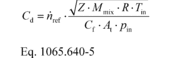Where: n ref = a reference molar flow rate.

(3) Determine Cf using one of the following methods:

(i) For CFV flow meters only, determine CfCFV from the following table based on your values for β and γ, using linear interpolation to find intermediate values:

Table 2 of § 1065.640-CfCFV Versus b and g for CFV Flow Meters

CfCFV
b gexh =
385
gdexh =
gair =
399
0.000 0.6822 0.6846
0.400 0.6857 0.6881
0.500 0.6910 0.6934
0.550 0.6953 0.6977
0.600 0.7011 0.7036
0.625 0.7047 0.7072
0.650 0.7089 0.7114
0.675 0.7137 0.7163
0.700 0.7193 0.7219
0.720 0.7245 0.7271
0.740 0.7303 0.7329
0.760 0.7368 0.7395
0.770 0.7404 0.7431
0.780 0.7442 0.7470
0.790 0.7483 0.7511
0.800 0.7527 0.7555
0.810 0.7573 0.7602
0.820 0.7624 0.7652
0.830 0.7677 0.7707
0.840 0.7735 0.7765
0.850 0.7798 0.7828

(ii) For any CFV or SSV flow meter, you may use the following equation to calculate Cf for each flow rate: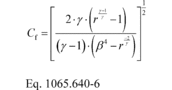Where: g = isentropic exponent. For an ideal gas, this is the ratio of specific heats of the gas mixture, Cp/Cv. r = pressure ratio, as determined in paragraph (c)(4) of this section. b = ratio of venturi throat to inlet diameters.

(4) Calculate r as follows:

(i) For SSV systems only, calculate rSSV using the following equation: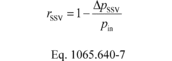Where: ΔpSSV = Differential static pressure; venturi inlet minus venturi throat.

(ii) For CFV systems only, calculate rCFV iteratively using the following equation: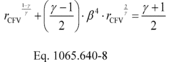(5) You may apply any of the following simplifying assumptions or develop other values as appropriate for your test configuration, consistent with good engineering judgment:

(i) For raw exhaust, diluted exhaust, and dilution air, you may assume that the gas mixture behaves as an ideal gas: Z = 1.

(ii) For raw exhaust, you may assume g = 1.385.

(iii) For diluted exhaust and dilution air, you may assume g = 1.399.

(iv) For diluted exhaust and dilution air, you may assume the molar mass of the mixture, Mmix, is a function only of the amount of water in the dilution air or calibration air, as follows: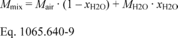Where: Mair = molar mass of dry air. xH2O = amount of H2O in the dilution air or calibration air, determined as described in § 1065.645. MH2O = molar mass of water. Example: Mair = 28.96559 g/mol xH2O = 0.0169 mol/mol MH2O = 18.01528 g/mol Mmix = 28.96559 · (1- 0.0169) + 18.01528 · 0.0169 Mmix = 28.7805 g/mol

(v) For diluted exhaust and dilution air, you may assume a constant molar mass of the mixture, Mmix, for all calibration and all testing as long as your assumed molar mass differs no more than ±1% from the estimated minimum and maximum molar mass during calibration and testing.

You may assume this, using good engineering judgment, if you sufficiently control the amount of water in calibration air and in dilution air or if you remove sufficient water from both calibration air and dilution air. The following table gives examples of permissible ranges of dilution air dewpoint versus calibration air dewpoint:

Table 3 of § 1065.640 - Examples of Dilution Air and Calibration Air Dewpoints at Which You May Assume a Constant Mmix

If calibration Tdew ( °C) is . . . assume the following constant Mmix (g/mol) . . . for the following ranges of Tdew ( °C) during emission tests a
dry 28.96559 dry to 18
0 28.89263 dry to 21
5 28.86148 dry to 22
10 28.81911 dry to 24
15 28.76224 dry to 26
20 28.68685 -8 to 28
25 28.58806 12 to 31
30 28.46005 23 to 34

a Range valid for all calibration and emission testing over the atmospheric pressure range (80.000 to 103.325) kPa.

(6) The following example illustrates the use of the governing equations to calculate Cd of an SSV flow meter at one reference flow meter value. Note that calculating Cd for a CFV flow meter would be similar, except that Cf would be determined from Table 2 of this section or calculated iteratively using values of b and g as described in paragraph (c)(2) of this section.

Example: n ref = 57.625 mol/s Z = 1 Mmix = 28.7805 g/mol = 0.0287805 kg/mol R = 8.314472 J/(mol · K) = 8.314472 (m 2 · kg)/(s 2 · mol · K) Tin = 298.15 K At = 0.01824 m 2 pin = 99.132 kPa = 99132.0 Pa = 99132 kg/(m·s 2) g = 1.399 b = 0.8 Δp = 2.312 kPa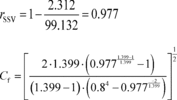Cf = 0.274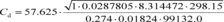Cd = 0.982

(d) SSV calibration. Perform the following steps to calibrate an SSV flow meter:

(1) Calculate the Reynolds number, Re#, for each reference molar flow rate, n ref, using the throat diameter of the venturi, dt. Because the dynamic viscosity, m, is needed to compute Re#, you may use your own fluid viscosity model to determine m for your calibration gas (usually air), using good engineering judgment. Alternatively, you may use the Sutherland three-coefficient viscosity model to approximate m, as shown in the following sample calculation for Re#: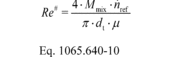Where, using the Sutherland three-coefficient viscosity model: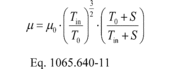Where: m0 = Sutherland reference viscosity. T0 = Sutherland reference temperature. S = Sutherland constant.

Table 4 of § 1065.640 - Sutherland Three-Coefficient Viscosity Model Parameters

Gas a m0 T0 S Temperature range within ± 2% error b Pressure limit b
kg/(m·s) K K K kPa
Air 1.716 · 10−5 273 111 170 to 1900 ≤ 1800
CO2 1.370 · 10−5 273 222 190 to 1700 ≤ 3600
H2 1.12 · 10−5 350 1064 360 to 1500 ≤ 10000
O2 1.919 · 10−5 273 139 190 to 2000 ≤ 2500
N2 1.663 · 10−5 273 107 100 to 1500 ≤ 1600

a Use tabulated parameters only for the pure gases, as listed. Do not combine parameters in calculations to calculate viscosities of gas mixtures.

b The model results are valid only for ambient conditions in the specified ranges.

Example: m0 = 1.716 · 10−5 kg/(m·s) T0 = 273 K S = 111 K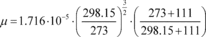µ = 1.838 · 10−5 kg/(m·s) Mmix = 28.7805 g/mol n ref = 57.625 mol/s dt = 152.4 mm = 0.1524 m Tin = 298.15 K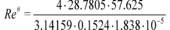Re# = 7.538·10 8

(2) Create an equation for Cd as a function of Re#, using paired values of the two quantities. The equation may involve any mathematical expression, including a polynomial or a power series. The following equation is an example of a commonly used mathematical expression for relating Cd and Re#: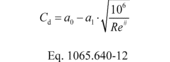(3) Perform a least-squares regression analysis to determine the best-fit coefficients for the equation and calculate SEE as described in § 1065.602.

(4) If the equation meets the criterion of SEE ≤ 0.5% · Cdmax, you may use the equation for the corresponding range of Re#, as described in § 1065.642.

(5) If the equation does not meet the specified statistical criterion, you may use good engineering judgment to omit calibration data points; however you must use at least seven calibration data points to demonstrate that you meet the criterion. For example, this may involve narrowing the range of flow rates for a better curve fit.

(6) Take corrective action if the equation does not meet the specified statistical criterion even after omitting calibration data points. For example, select another mathematical expression for the Cd versus Re# equation, check for leaks, or repeat the calibration process. If you must repeat the calibration process, we recommend applying tighter tolerances to measurements and allowing more time for flows to stabilize.

(7) Once you have an equation that meets the specified statistical criterion, you may use the equation only for the corresponding range of Re#.

(e) CFV calibration. Some CFV flow meters consist of a single venturi and some consist of multiple venturis, where different combinations of venturis are used to meter different flow rates. For CFV flow meters that consist of multiple venturis, either calibrate each venturi independently to determine a separate discharge coefficient, Cd, for each venturi, or calibrate each combination of venturis as one venturi. In the case where you calibrate a combination of venturis, use the sum of the active venturi throat areas as At, the square root of the sum of the squares of the active venturi throat diameters as dt, and the ratio of the venturi throat to inlet diameters as the ratio of the square root of the sum of the active venturi throat diameters (dt) to the diameter of the common entrance to all the venturis. (D). To determine the Cd for a single venturi or a single combination of venturis, perform the following steps:

(1) Use the data collected at each calibration set point to calculate an individual Cd for each point using Eq. 1065.640-4.

(2) Calculate the mean and standard deviation of all the Cd values according to Eqs. 1065.602-1 and 1065.602-2.

(3) If the standard deviation of all the Cd values is less than or equal to 0.3% of the mean Cd, use the mean Cd in Eq. 1065.642-4, and use the CFV only up to the highest venturi pressure ratio, r, measured during calibration using the following equation: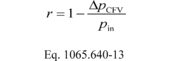Where: ΔpCFV = Differential static pressure; venturi inlet minus venturi outlet.

(4) If the standard deviation of all the Cd values exceeds 0.3% of the mean Cd, omit the Cd value corresponding to the data point collected at the highest r measured during calibration.

(5) If the number of remaining data points is less than seven, take corrective action by checking your calibration data or repeating the calibration process. If you repeat the calibration process, we recommend checking for leaks, applying tighter tolerances to measurements and allowing more time for flows to stabilize.

(6) If the number of remaining Cd values is seven or greater, recalculate the mean and standard deviation of the remaining Cd values.

(7) If the standard deviation of the remaining Cd values is less than or equal to 0.3% of the mean of the remaining Cd, use that mean Cd in Eq. 1065.642-4, and use the CFV values only up to the highest r associated with the remaining Cd.

(8) If the standard deviation of the remaining Cd still exceeds 0.3% of the mean of the remaining Cd values, repeat the steps in paragraph (e)(4) through (8) of this section.

[79 FR 23785, Apr. 28, 2014, as amended at 81 FR 74172, Oct. 25, 2016]